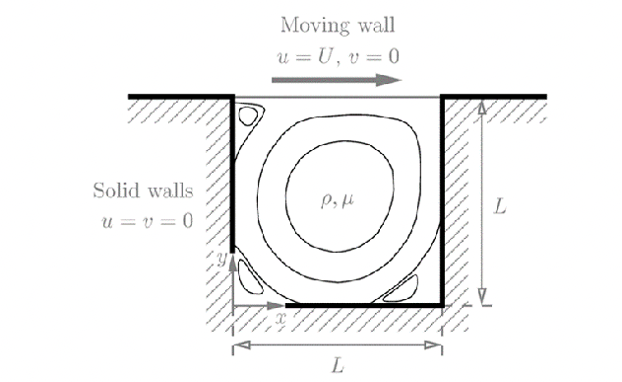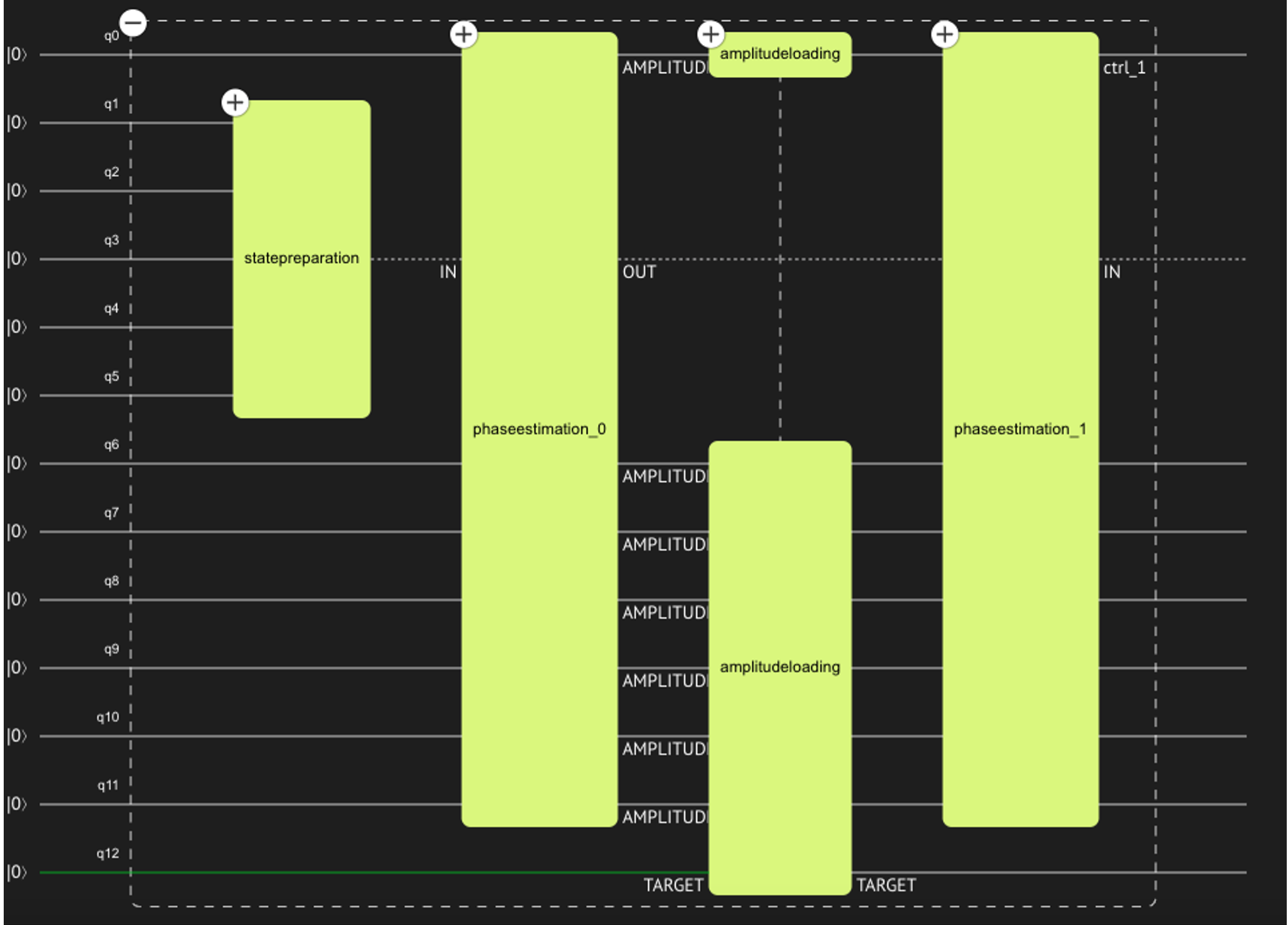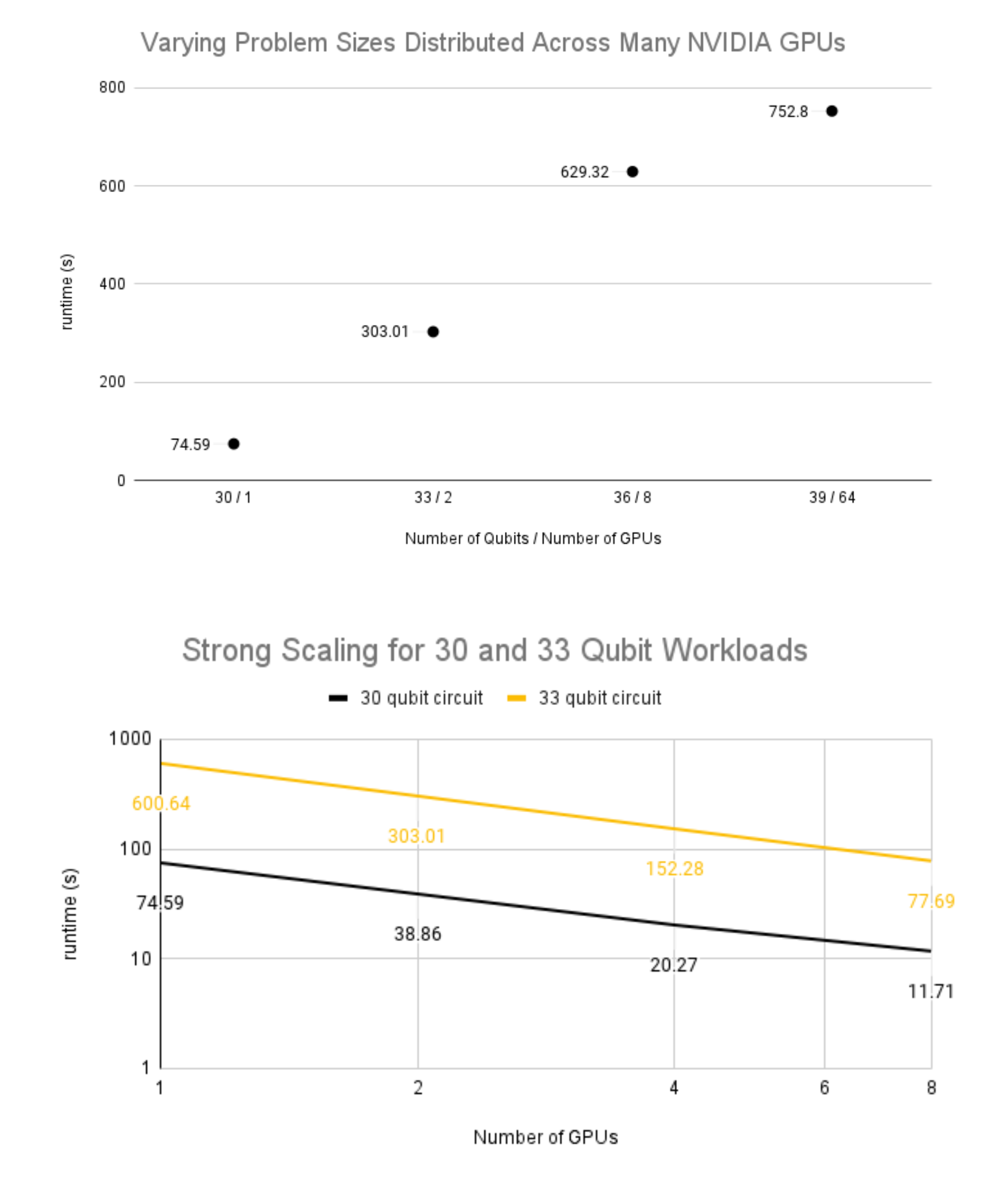Blog

# Pioneering Quantum Computing in Computational Fluid Dynamics: A Collaboration Between Classiq and NVIDIA

22
May
,
2023

In the realm of Computational Fluid Dynamics (CFD), the complexities of numerical simulations involving fluid and gas motion are immense. These state-of-the-art numerical schemes often involve mapping physical problems into a blend of non-linear and linear equations that require intricate numerical solutions. As we move into the future, quantum computations are poised to play an essential role in addressing these challenges.An example of a lid driven cavity, one of the main test cases for CFD. Such a test case can be examined efficiently through the use of a quantum linear solver such as HHL (image from Lapworth, arXiv preprint arXiv:2209.07964 (2022)).

A shining example of this potential is the HHL algorithm, named after its creators Harrow, Hassidim, and Lloyd. This fundamental quantum algorithm is designed to solve linear sets of equations and holds the promise of speedier computations than its classical counterparts. The application of the HHL algorithm within a hybrid CFD scheme opens up exciting possibilities. The non-linear and linear components of the problem can be tackled by classical and quantum hardware respectively, creating an efficient and powerful solution framework.An HHL circuit generated by the Classiq platform. This circuit is built using four quantum function blocks. The accuracy of this quantum linear solver depends on the depth and number of extra qubits employed in the Quantum Phase Estimation block.

However, implementing the HHL algorithm in the context of large CFD problems and on quantum hardware is not without its challenges. It necessitates a meticulous exploration of quantum circuit design and quality on quantum emulators. As the HHL algorithm is approximated, the level of accuracy is influenced by various factors including different quantum function implementations, the number of qubits, and circuit depth. The Classiq platform offers an optimal space to navigate this exploration with ease.

Alongside this exploration, there is a need for efficient quantum emulators that can verify the functionality and accuracy level of quantum circuits. These simulators must have the capacity to handle quantum circuits with a large number of qubits. This will allow for the examination of various approximation levels and facilitate the addressing of significant hydrodynamic problems. Timing is a crucial aspect of this process. It is essential to evaluate the quantum part's functionality within a full hybrid CFD scheme, where the input and output of a quantum linear solver are frequently transferred from a classical part.NVIDIA simulator results showing execution times for varying numbers of qubits and varying numbers of GPUs. Results show that increasing the number of GPUs employed in the quantum simulator with a fixed number of qubits can reduce computation time by an order of magnitude.

In this quest for quantum innovation, Classiq is excited to collaborate with NVIDIA. Together, we're leveraging the power of the HHL quantum algorithm in CFD to overcome the challenges inherent in the domain. As we delve into this pioneering era of quantum-enhanced solutions, we anticipate a transformative impact across a range of industries. The journey ahead is filled with untapped potential, and we are eager to explore the breakthroughs that await us.In the realm of Computational Fluid Dynamics (CFD), the complexities of numerical simulations involving fluid and gas motion are immense. These state-of-the-art numerical schemes often involve mapping physical problems into a blend of non-linear and linear equations that require intricate numerical solutions. As we move into the future, quantum computations are poised to play an essential role in addressing these challenges.An example of a lid driven cavity, one of the main test cases for CFD. Such a test case can be examined efficiently through the use of a quantum linear solver such as HHL (image from Lapworth, arXiv preprint arXiv:2209.07964 (2022)).

A shining example of this potential is the HHL algorithm, named after its creators Harrow, Hassidim, and Lloyd. This fundamental quantum algorithm is designed to solve linear sets of equations and holds the promise of speedier computations than its classical counterparts. The application of the HHL algorithm within a hybrid CFD scheme opens up exciting possibilities. The non-linear and linear components of the problem can be tackled by classical and quantum hardware respectively, creating an efficient and powerful solution framework.An HHL circuit generated by the Classiq platform. This circuit is built using four quantum function blocks. The accuracy of this quantum linear solver depends on the depth and number of extra qubits employed in the Quantum Phase Estimation block.

However, implementing the HHL algorithm in the context of large CFD problems and on quantum hardware is not without its challenges. It necessitates a meticulous exploration of quantum circuit design and quality on quantum emulators. As the HHL algorithm is approximated, the level of accuracy is influenced by various factors including different quantum function implementations, the number of qubits, and circuit depth. The Classiq platform offers an optimal space to navigate this exploration with ease.

Alongside this exploration, there is a need for efficient quantum emulators that can verify the functionality and accuracy level of quantum circuits. These simulators must have the capacity to handle quantum circuits with a large number of qubits. This will allow for the examination of various approximation levels and facilitate the addressing of significant hydrodynamic problems. Timing is a crucial aspect of this process. It is essential to evaluate the quantum part's functionality within a full hybrid CFD scheme, where the input and output of a quantum linear solver are frequently transferred from a classical part.NVIDIA simulator results showing execution times for varying numbers of qubits and varying numbers of GPUs. Results show that increasing the number of GPUs employed in the quantum simulator with a fixed number of qubits can reduce computation time by an order of magnitude.

In this quest for quantum innovation, Classiq is excited to collaborate with NVIDIA. Together, we're leveraging the power of the HHL quantum algorithm in CFD to overcome the challenges inherent in the domain. As we delve into this pioneering era of quantum-enhanced solutions, we anticipate a transformative impact across a range of industries. The journey ahead is filled with untapped potential, and we are eager to explore the breakthroughs that await us.

## About "The Qubit Guy's Podcast"

Hosted by The Qubit Guy (Yuval Boger, our Chief Marketing Officer), the podcast hosts thought leaders in quantum computing to discuss business and technical questions that impact the quantum computing ecosystem. Our guests provide interesting insights about quantum computer software and algorithm, quantum computer hardware, key applications for quantum computing, market studies of the quantum industry and more.